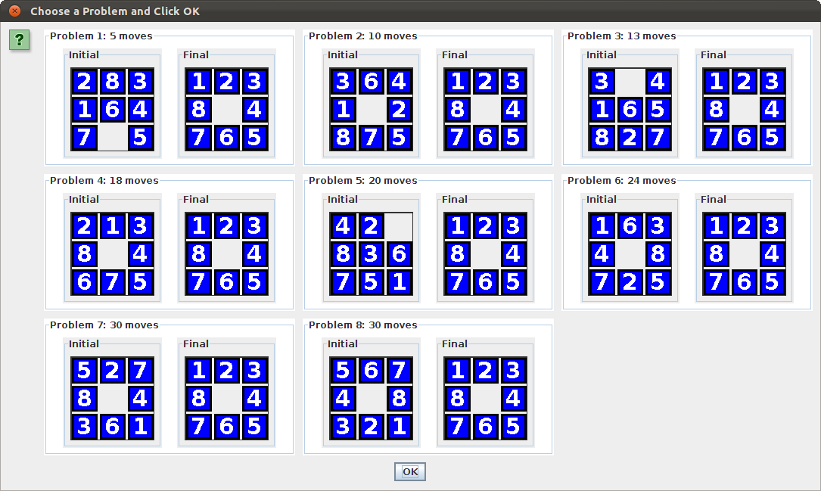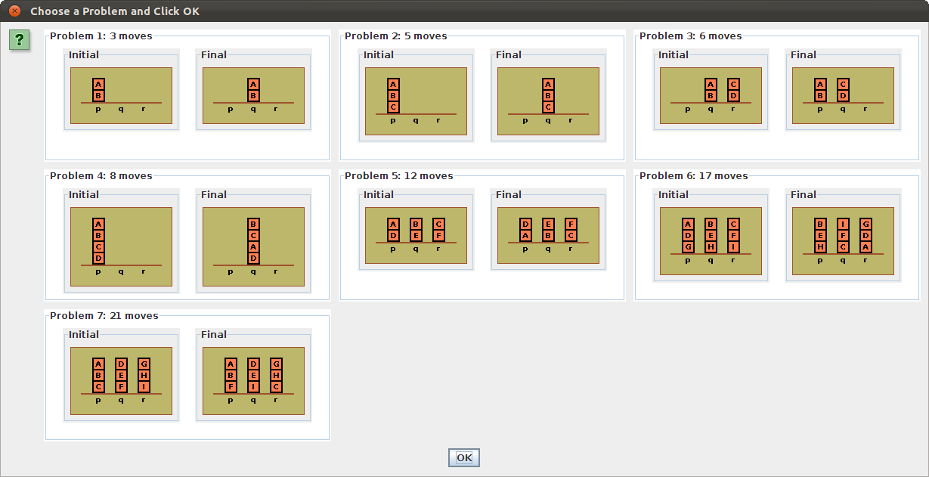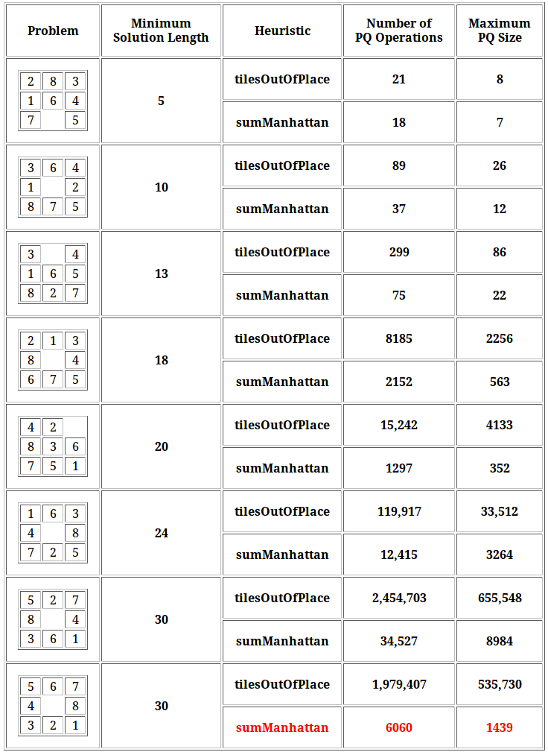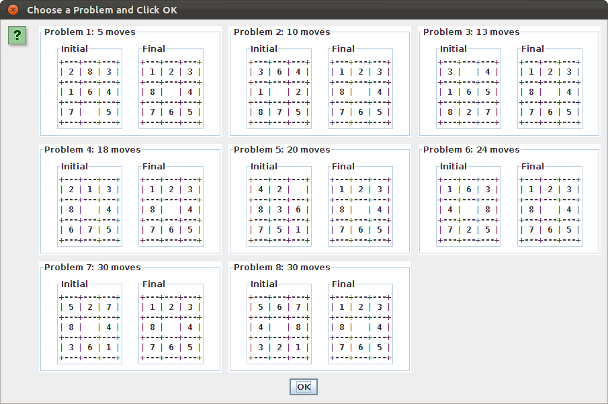This assignment has three main parts:
• Add the Blocks World problem to the problems already supported by the problem solving framework
• All classes except BlockCanvas are provided for you
• Modify the framework from the previous assignment so that A* search replaces breadth-first or depth-first search of the problem's state space
• Add heuristics to the 8-Puzzle and Blocks World problems so that optimal solutions are efficiently found
Click Program Behavior to the left to see how your program might look upon completion.

For A* search you will need to thoroughly understand the Informed State Space Search lecture notes.

• An applet showing final behavior of the program is under Demonstration Applet to the left.
• Note that for the puzzle and block problems, upon RESET the user can choose among different problem benchmarks.
• The benchmark tests are shown under Puzzle Problems and Blocks Problems to the left.
• The code to implement the problem chooser is given to you (see How To Proceed).• The GUI displays the final (goal) state as well as the current state
• The choice of depth-first search or breadth-first search is eliminated.
• Clicking SOLVE invokes an A* search from the current state, so the solution found must be optimal (if the problem's heuristic does not overestimate)
• Search statistics show priority queue rather than double-ended queue operations
• The bridge and water jug problems can have trivial heuristics (h = 0) due to small search spaces
• The 8-puzzle and blocks world problems must have non-trivial heuristics that do not overestimate
• Clicking RESET for the 8-puzzle or blocks world allows the user to select from a number of benchmark problems
Before you start, use NetBeans to copy your CS_2511_Solve project from the previous assignment to a new NetBeans project called CS_2511_Astar (right click the project node in the Projects window and select Copy...).

In this section we describe:

• How to implement A*
• Required changes to the framework
• Use of the problem chooser
• The blocks world domain
For details see Informed State Space Search.

In the framework:

• Modify Problem.search to use a priority queue rather than a double-ended queue
• Add a getHeuristic method to the State interface
In the framework applications:
• For BridgeState and WaterJugState, getHeuristic can return 0
• For a PuzzleState heuristic see Test 1 and Test 2 (to the left)
• Your BlockState heuristic must wait till you've added the Blocks World (see to the left)
When your A* search is working, you should get results comparable to these:In order to display the final state in the GUI:
• The Problem class must store the final state along with the current state, which means:
• The Problem class must have a getter and setter for the final state, so that:
• The constructors for the extending classes (BridgeProblem, etc.) can provide the final state to the parent.
• Also, the GUI constructor needs a third argument to receive the final state canvas (which can be obtained from the problem)
In order to display the Problem Chooser (see to the left):
• The Problem class must store lists of initial states and final states, and (optionally) lists of initial canvases and final canvases, which means:
• The Problem class must have getters and setters for each of these lists, so that:
• The constructors for the extending classes (BridgeProblem, etc.) can provide these lists to the parent.
In order to compute the required statistics:
• Initialize counters for queueOps, queueSize, and maxQueueSize before the search
• Increment queueOps each time PQ is added to or removed from
• Decrement queueSize each time PQ is removed from
• Increment queueSize each time PQ is added to, and set maxQueueSize to max(queueSize, maxQueueSize)
• Display queueOps and maxQueueSize in the GUI upon search completion.
This section shows how to use the constructor that creates a dialog with string depictions of problem states.
The following code might be from the PuzzleProblem constructor:
```        PuzzleState start1 =
new PuzzleState('2', '8', '3', '1', '6', '4', '7', ' ', '5'); // 5 moves

PuzzleState start2 =
new PuzzleState('3', '6', '4', '1', ' ', '2', '8', '7', '5'); // 10 moves

PuzzleState start3 =
new PuzzleState('3', ' ', '4', '1', '6', '5', '8', '2', '7'); // 13 moves

PuzzleState start4 =
new PuzzleState('2', '1', '3', '8', ' ', '4', '6', '7', '5'); // 18 moves

PuzzleState start5 =
new PuzzleState('4', '2', ' ', '8', '3', '6', '7', '5', '1'); // 20 moves

PuzzleState start6 =
new PuzzleState('1', '6', '3', '4', ' ', '8', '7', '2', '5'); // 24 moves

PuzzleState start7 =
new PuzzleState('5', '2', '7', '8', ' ', '4', '3', '6', '1'); // 30 moves

PuzzleState start8 =
new PuzzleState('5', '6', '7', '4', ' ', '8', '3', '2', '1'); // 30 moves

PuzzleState finalState =
new PuzzleState('1', '2', '3', '8', ' ', '4', '7', '6', '5');

List<State> initialStates = new ArrayList<State>();
List<State> finalStates = new ArrayList<State>();
List<Integer> moveCounts = new ArrayList<Integer>();

setInitialStates(initialStates);
setFinalStates(finalStates);
setMoveCounts(moveCounts);
```
The following framework code would pop up the dialog:
```        new ProblemChooser(problem.getInitialStates(),
problem.getFinalStates(),
problem.getMoveCounts());
```This section shows how to use the constructor that creates a dialog with canvas depictions of problem states.
In addition to the setup code for the first constructor, add the following:
```        List<Canvas> initialCanvases = new ArrayList<Canvas>();
List<Canvas> finalCanvases = new ArrayList<Canvas>();

setInitialCanvases(initialCanvases);
setFinalCanvases(finalCanvases);
```
The following framework code would pop up the dialog:
```        new ProblemChooser(problem.getInitialStates(),
problem.getFinalStates(),
problem.getMoveCounts(),
problem.getInitialCanvases(),
problem.getFinalCanvases());
```This section describes the blocks world problem and a package of blocks world classes that can be plugged into the problem solving framework.

You will need to add a BlockCanvas class for use with the GUI.

Here is a zipped folder containing a package called blocks that you should install under Source Packages in your CS_2511_Astar NetBeans project: blocks.zip.

If NetBeans complains that the BlockState constructors do not match up with SimpleVertex in the graph package, add the following to SimpleVertex:

```    public SimpleVertex() {
this("");
}

```
You should be able to run the BlockConsole.java file and get behavior like that in this applet:

When your program is working correctly you will submit your entire Netbeans project, but first:
• To double-check that everything is in order, click Build on the menu bar and select Clean and Build Main Project.
• Run the framework.test.TestFrame class to be sure all four problems work.